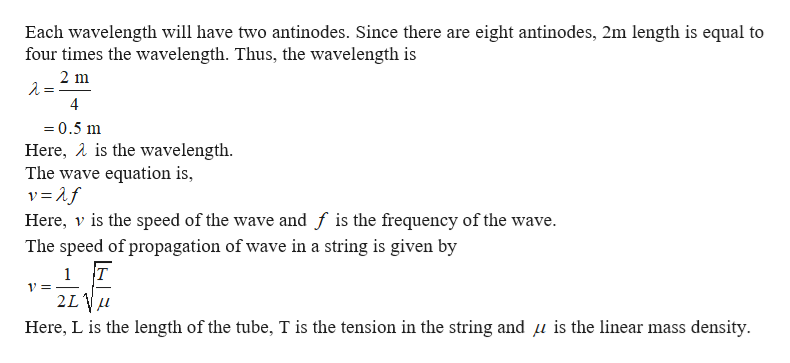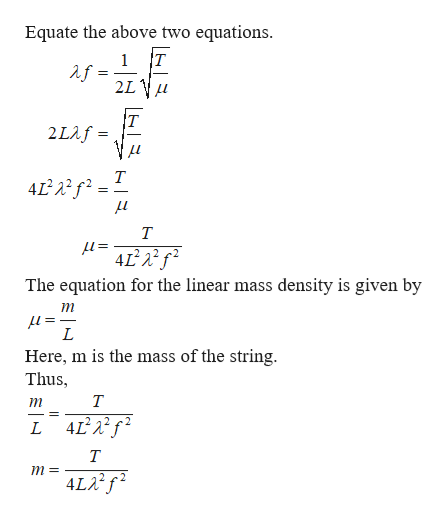# When a string that is 2m long is connected between two supports and vibrated at 176 Hz it is observed to produce 8 antinodes. If the tension on the string is 77.5 N what would the total mass of the string be?

Question
1 views
When a string that is 2m long is connected between two supports and vibrated at 176 Hz it is observed to produce 8 antinodes. If the tension on the string is 77.5 N what would the total mass of the string be?
check_circle

Step 1help_outlineImage TranscriptioncloseEach wavelength will have two antinodes. Since there are eight antinodes, 2m length is equal to four times the wavelength. Thus, the wavelength is 2 m 4 =0.5 m Here, A is the wavelength. The wave equation is, v=2f Here, v is the speed of the wave and f is the frequency of the wave The speed of propagation of wave in a string is given by 1 T 2L Here, L is the length of the tube, T is the tension in the string and u is the linear mass density fullscreen
Step 2help_outlineImage TranscriptioncloseEquate the above two equations. T 1 2LV 2Laf= т Т =1 4L2 The equation for the linear mass density is given by т = 1 Here, m is the mass of the string. Thus L L 4 т m= 4LA fullscreen

### Want to see the full answer?

See Solution

#### Want to see this answer and more?

Solutions are written by subject experts who are available 24/7. Questions are typically answered within 1 hour.*

See Solution
*Response times may vary by subject and question.
Tagged in

### Wave Motion